# Calculator multiplication of mixed numbers

The calculator performs basic and advanced operations with mixed numbers, fractions, integers, decimals. Mixed fractions are also called mixed numbers. A mixed fraction is a whole number and a proper fraction combined, i.e. one and three-quarters. The calculator evaluates the expression or solves the equation with step-by-step calculation progress information. Solve problems with two or more mixed numbers fractions in one expression.

### 33/4 * 22/5 = 9/1 = 9

Spelled result in words is nine.

### Calculation steps

1. Conversion a mixed number 3 3/4 to a improper fraction: 3 3/4 = 3 3/4 = 3 · 4 + 3/4 = 12 + 3/4 = 15/4

To find a new numerator:
a) Multiply the whole number 3 by the denominator 4. Whole number 3 equally 3 * 4/4 = 12/4
b) Add the answer from previous step 12 to the numerator 3. New numerator is 12 + 3 = 15
c) Write a previous answer (new numerator 15) over the denominator 4.

Three and three quarters is fifteen quarters
2. Conversion a mixed number 2 2/5 to a improper fraction: 2 2/5 = 2 2/5 = 2 · 5 + 2/5 = 10 + 2/5 = 12/5

To find a new numerator:
a) Multiply the whole number 2 by the denominator 5. Whole number 2 equally 2 * 5/5 = 10/5
b) Add the answer from previous step 10 to the numerator 2. New numerator is 10 + 2 = 12
c) Write a previous answer (new numerator 12) over the denominator 5.

Two and two fifths is twelve fifths
3. Multiple: 15/4 * 12/5 = 15 · 12/4 · 5 = 180/20 = 9 · 20/1 · 20 = 9
Multiply both numerators and denominators. Result fraction keep to lowest possible denominator GCD(180, 20) = 20. In the following intermediate step, cancel by a common factor of 20 gives 9/1.
In other words - fifteen quarters multiplied by twelve fifths = nine.

## Mixed fractions in word problems:

• Muffins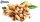One batch of walnut muffins uses 1 1/3 cups of walnuts. How many cups of walnut are needed to make 3 3/4  batches of muffins?
• The product 3The product of two numbers is 15 ⁵/₆. If one of the numbers is 5 ²/₃, find the other.
• Judy baking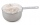Judy is baking a cake and has 1 3/4 cups of flour. The recipe for the cake called for 1 1/3 times this amount. How many cups of flour does Judy need?
• Caroline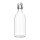Caroline collected 3 ¼ bags of recycling to take to the recycling center. ⅓ of the bags are glass bottles. How many bags will be filled with glass bottles?
• Jim collectedJim collected 5 bins of glass bottles to recycle. Lucia collected 3 1/5 times as many bins as Jim. How many bins of bottles did Lucia collect?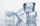Miriam is making 3 1/2 dozen cookies. If 2/7 of the cookies have icing, how many dozen cookies have icing?
• A burger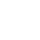The water starts with 41 1/4 cups of water how much water is left after ten 3 5/8 Scoops of water are removed?
• The product 2The product of two functions is 10. If one of them is 2 1/3, find the other one.
• Twelve eggs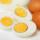A dozen eggs cost 80 cents . how much will 3½ dozen eggs cost?
• Numbers divisionWith what number should be divided mixed number 2 3/4 to get 11/12?
• Eight 9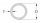Eight pipes are 2¼ meter long . what is the total length of the eight pipes?
• Equivalent fractions 2Write the equivalent multiplication expression. 2 1/6÷3/4
• Tablespoon of saltMrs. Monika uses 3/4 tablespoons of salt for every kilogram of ground pork. How many tablespoons of salt will she use for 3 1/2 kilograms of ground pork?
• JefferyJeffery is painting a wall the width of the wall is 9 feet the length of the was is 8 2/3 feet what is the area of the wall Jeffery wants to paint?
• Juila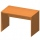Juila has a rectangular table that is 3 1/2 feet long and 1 3/4 feet wide. What is the area of Juila's table?
• Marbles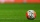Dave had 40 marbles. Junjun has 2 1/5 more than Dave’s marbles. How many marbles do they have altogether?
• Oil change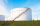A container holds 1/4 gallon of oil. Jack is changing the oil in his vehicles and needs 11 containers. How many gallons of oil does Jack need?